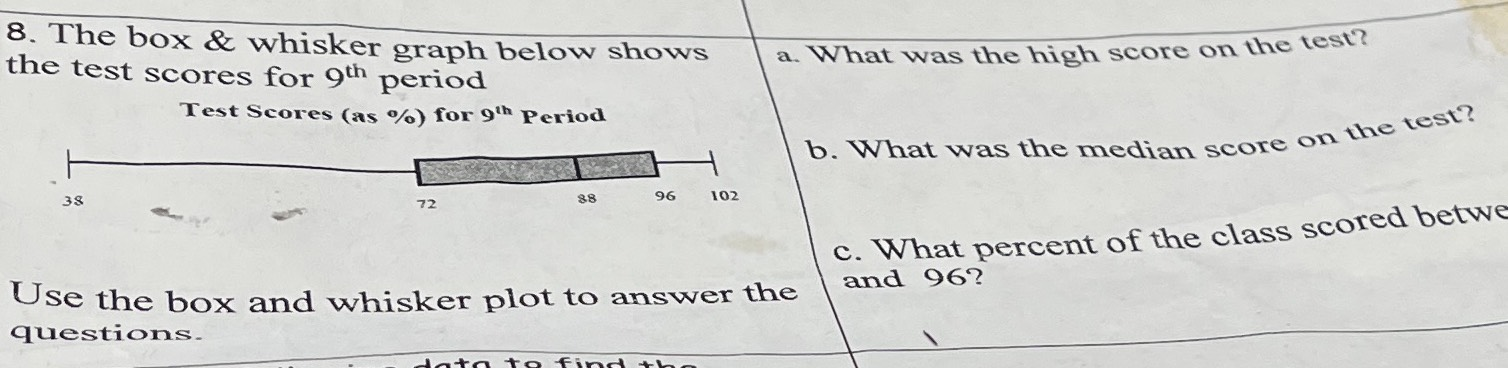### ¿Todavía tienes preguntas de matemáticas?

Pregunte a nuestros tutores expertos
Algebra
Pregunta8. The box  & whisker graph below shows the test scores for 9$$^{th}$$ period. Use the box and whisker plot to answer the questions.  a. What was the high score on the test? b. What was the median score on the test?C. What percent of the class scored between and $$96 ?$$